Courses

# Testing Of DC Series Motors Electrical Engineering (EE) Notes | EduRev

## Electrical Engineering (EE) : Testing Of DC Series Motors Electrical Engineering (EE) Notes | EduRev

The document Testing Of DC Series Motors Electrical Engineering (EE) Notes | EduRev is a part of the Electrical Engineering (EE) Course Electrical Machines.
All you need of Electrical Engineering (EE) at this link: Electrical Engineering (EE)

Testing of DC Series Motors
(Refer Slide Time: 00:23)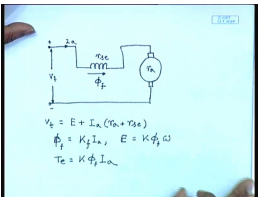Few classes we have derived the torque speed characteristics of a DC series motor. We can write induced voltage E plus I a. If we assume that the machine is unsaturated, then phi f equal to some K f into I a for series machine. And E of course is K phi f omega; also torque equal to K phi f I a.

(Refer Slide Time: 02:02)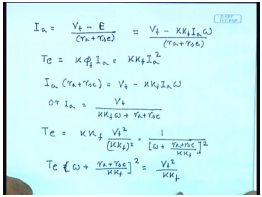From this we derived that, so from here we get r a plus r a c and torque I a square. So, this is r.

(Refer Slide Time: 04:58)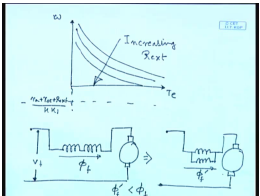This torque speed characteristics when plotted looks somewhat like this. We have also seen that these torque speed characteristics can be manipulated by let us say changing r a plus r s e by incorporating additional resistance in the armature circuit. So, with added resistance this point which is minus r a plus r a c plus r external by K k f goes down, and with increasing r external we can obtain characteristics like this. Another way to vary it is to vary the value of K k f; that can be done by series parallel combination of field coils.

For example, if you have all field coils may be in series, from here you can reconnect these field coils let us say in parallel; in this way also you can change the torque speed characteristics of the. This has the effect of reducing the field flux. If this was phi f and this was phi f dash, then phi f dash will be less than phi f, and the torque speed characteristics will shift upwards. Let us see by a few examples how this is done.

(Refer Slide Time: 07:28)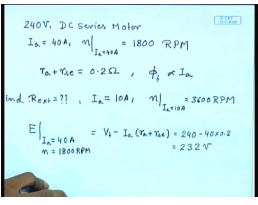Consider a 240 volt DC series motor used for lift drive with armature current of I a equal to 40 amperes; the speed I a equal to 40 amperes. This comes to 1800 RPM. It is given that the sum of the armature resistance and series field resistance is 0.2 ohm, and also the machine is unsaturated; that is the field flux is proportional to I a. Now let us try to find out how much R external is necessary if at a load current armature current I a equal to 10 ampere, we want n to be 3600 RPM.

So, for this let us try to find out what is E when I a equal to 40 ampere and n equal to 1800 RPM, and this can be solved from V t minus I a r a plus r s E. Because we have been told that without any external resistance when I a equal 40 ampere n equal to 1800 RPM. This comes to 240 minus 40 into 0.2. This is equal to 232 volts.

(Refer Slide Time: 10:37)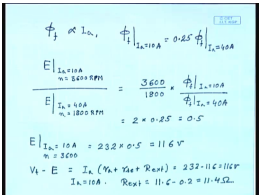Since, phi f is proportional to I a we know phi f at I a equal to 10 ampere equal to 0.25 times phi f at I a equal to 40 amperes. Also the ratio of E at I a equal to 10 ampere n equal to 3600 RPM divided by E at I a equal to 40 ampere n equal to 1800 RPM equal to 3600 by 1800 into phi f at I a equal to 10 amperes divided by phi f at I a equal to 40 amperes. So, this is equal to 2 into 0.25 equal to 0.5, and we know that this quantity to be 232. So, E at I a equal to 10 amperes n equal to 3600 equal to 232 into 0.5. This comes to 116 volts.

Therefore, V t minus E equal to I a into r a plus r a c plus r external equal to 232 minus 116 equal to 116 volt. I a equal to 10 amperes from which we get R external equal to 11.6 minus 0.2 equal to 11.4 ohm. So, we will have to connect an external resistance of 11.4 ohm if we want to restrict the speed to 3600 RPM when the armature current is 10 ampere.

(Refer Slide Time: 14:03)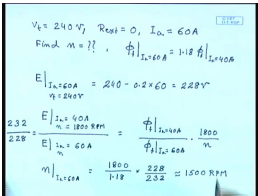Let us now try to find out if we do not connect any external resistance and increase the load armature current to 60 ampere when the machine is connected to 240 volts; that is V t equal to 240 volts R external equal to zero and the loading is such that the armature current is 60 ampere. What will be the speed, find n? It is also given that phi f at I a equal to 60 ampere equal to 1.18 times phi f at I a equal to 40 ampere. So, for that let us first find what will be the induced voltage E. This is 240 minus 0.2 into 60 equal to 228 volts, and 40 ampere when n equal to 1800 RPM divided by E at I a equal to 60 ampere and n. This is phi f at I a equal to 40 ampere divided by phi f at I a equal to 60 ampere into 1800 by n.

But this is equal to 232 by 228; therefore, from here we get n at I a equal to 60 ampere equal to 1800 by 1.18 into. It is approximately 1500 RPM. So, this is how you can control the speed of a DC series motor by connecting external resistance. Let us look at some calculation regarding the other method of speed control; that is when you connect the field windings in series parallel combination.

(Refer Slide Time: 17:43)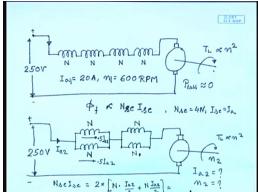So, let us take a fourfold DC series motor. It had four series connected field winding; all windings are connected in series. Say, the rated terminal voltage is 250 volts. Each of this let us say have a turn of N; with I a equal to 20 ampere the speed n is 600 RPM. The load torque T L on the shaft is proportional to n square. Assume that all losses is unnegligible and also the magnetic circuit unsaturated; that is phi f is proportional to field ampere turn; that is the effective series number of turns and the current through the series coil. In this particular case N s e equal to 4 n and I s e equal to I a.

However, it does not always have to be the case. Now this all series connected coil I now change in this fashion. I have two coils connected in parallel in a group and then another two coils connected in parallel in another group. And these two groups are now connected in series with the same load torque. Find out what will be the armature current and the speed in this case? Here I a 1 is 20 ampere n 1 equal to let us say this is connection 1; I a 1 is 20 ampere, n 1 equal to 600 RPM, and let us say now the speed is n 2.

So, we are supposed to find out what will be I a 2 and what will be N 2? Obviously, in this case assuming that the coils are identical the current through each of the coil is only half the armature current. So, here the net field ampere turns N s e I s e equal to 2 into I a 2 by 2 plus N into I a 2 by 2. This is 2 N I a 2. Here it was 4 N I a 1.

(Refer Slide Time: 23:27)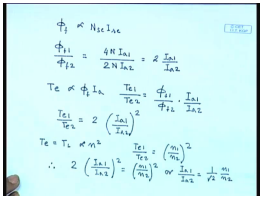Therefore, the ratio of field fluxes since phi f is proportional to N s e I s e phi f 1 by phi f 2, and this should be equal to 4 N I a 1 divided by 2 N I a 2. This is equal to two times I a 1 by I a 2. Also the electromagnetic torque is proportional to phi f I a; therefore, the torque in the first case T e 1 divided by T e 2 will be equal to phi f 1 by phi f 2 into I a 1 by I a2. Therefore, T e 1 by T e 2 equal to two times phi a 1 by phi a 2 whole square, but in steady state T e equal to T L which is proportional to n square. Therefore, T e 1 by T e 2 is equal to n 1 by n 2 whole square. So, two times I a 1 by I a 2 whole square equal to n 1 by n 2 whole square or I a 1 by I a 2 equal to 1 by root 2 n 1 by n 2. Now let us look at the other side.

(Refer Slide Time: 26:18)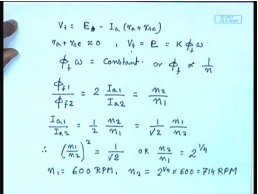For any DC motor we know V t equal to E t minus I a r a plus r s e. Now in this case all losses are negligible. So, r a plus r s e is approximately zero. So, V t equal to E equal to K phi omega. Since, V t remains constant we can say phi f omega equal to constant or phi f proportional to 1 by RPM. So, we have phi f 1 by phi f 2 equal to which we have already found out to be two times I a 1 by I a 2. This is also equal to n 2 by n 1.

Therefore, we have another relationship I a 1 by I a 2 equal to half n 2 by n 1, and we have also seen this is equal to 1 by root 2 n 1 by n 2. So, we have n 1 by n 2 square equal to 1 by root 2 or n 2 by n 1 equal to 2 to the power one-fourth. Now we know n 1 equal to 600 PRM; therefore, from here we will find out n 2 to be equal to 2 to the power oneforth into 600 into 714 RPM.

(Refer Slide Time: 29:15)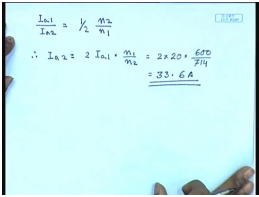How about I a 1 and I a 2? So, I a 2 equal to two times I a 1 into n 1 by n 2. It becomes to 2 into I a 1 is 20 amperes into 600 by 714. This comes to 33.6 amperes. We see that with a given load characteristics by connecting the configurations in changing the configurations of the field winding, we can change the speed of the DC series motor. However, it should be noted that we can obtain only discrete values of speed; the speed cannot be changed in a continuous fashion. In order to have a continuous speed change, we should use either a continuously variable armature resistance or if we want efficient operation this DC series motor can be fed from a variable DC voltage source such as a chopper.

Like DC shunt motors it is also necessary to find out experimentally the losses and efficiency of DC series motors. In case of DC shunt motor, we have seen there are two tests 1 is called the Swinburne test which is basically a no load test which find out the no load rotational losses and constant losses. And then there was Hopkinson test which is a regenerative test to find out the efficiency and losses at different loading of the DC machine. We would like to do similar tests on a DC series motor; however, one problem is that a DC series motor an equivalent test Swinburne test is not possible, because at no load the DC series motor speed tends to become very high. So, it is not advisable to operate the motor at no load; therefore, we have to find out the losses by load testing only. Here also there are some difficulties in doing back to back test.

(Refer Slide Time: 32:19)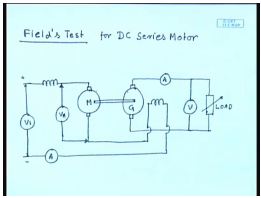So, the machine has to be actually loaded to do this test, and the test is called the field test. Field’s test like Hopkinson test for shunt motors; here also you need two identical DC series motors which are mechanically coupled. One of them acts as a motor; another act as a generator, but the difference is the generator does not feed the motor. There is no back to back connection; rather, the generator feeds an external load variable load.

The motor field winding and the generator field winding are connected in series and both in series with the motor armature. You measure the terminal voltage here and also the voltage across the motor terminal as well as the armature current of the motor, the armature current of the generator and the load voltage. So, the set these are two identical DC series motor and since their field windings are connected in series the iron loss and the no load rotational loss of both the motors are assumed to be same.

(Refer Slide Time: 35:18)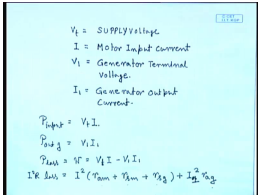The readings that we take are V t equal to supply voltage, and this is I the motor supply current. This is I 1 the generator output current, and this is V 1 the load voltage, generator output current. Now the power input to the set equal to V t I and power output of the generator equal to V 1 I 1. So, total loss equal to W is V t I minus V 1 I 1. I square R loss is given by I square into motor armature resistance plus motor series field resistance plus generator series field resistance plus generator armature copper loss. Now these resistances can be individually found by voltage drop method of those components.

(Refer Slide Time: 38:41)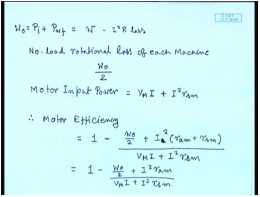So, the no load iron and rotational losses P I plus P w friction and windage losses; this is equal to total loss W minus I square R loss. So, this is W 0, no load rotational losses. So, no load rotational losses, since, these are identical machine we are saying no load rotational losses of each machine. This will be W 0 by 2. Motor input power is this V m multiplied by V M I. So, motor efficiency will be V M I plus of course, I square r s m 1 minus W 0 by 2 plus I square. This can also be written as, what about the generator input? This we can say nu motor.

(Refer Slide Time: 42:40)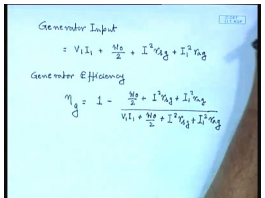The generator input, this is equal to V 1 I 1 plus this is the output of the generator W 0 by 2; this is the no load rotational losses plus I square r s g. This is the series field loss of the generator plus r a g, this is the armature copper loss of the generator. So, generator efficiency nu g equal to 1 minus losses in the generator divided by the total input of the generator. So, this is how we can find out the losses and efficiency of the individual machines from field test. Let us look at an example to see how exactly this is done. For that let us consider field test on two identical DC series motor.

(Refer Slide Time: 45:09)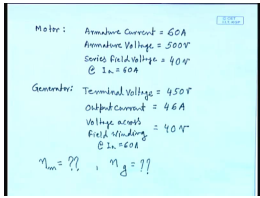For the motor the data is like this. The armature current into 60 ampere, armature voltage equal to 500 volts, series field voltage at I a equal to 60 ampere. This comes to 40 volts. For the generator, terminal voltage is 450 volts, output current equal to 46 amperes and field voltage across field winding again at I a equal to 60 amperes. This comes to also 40 volt. So, find out what is motor efficiency, and what is generator efficiency from this data. Let us see how we use this data to find the motor and the generator efficiency.

(Refer Slide Time: 47:53)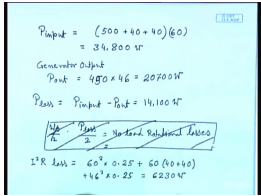So, what is the total power input to the set p input? This is equal to the voltage across the motor armature plus voltage across the motor series field plus voltage across the generator series field into the armature current 60 ampere. This comes to 34800 watts. Generator output p out; this is generator terminal voltage 450 volt into generator output current 46 amperes. This comes to 20700 watts. So, p loss equal to p input minus p out. This comes to 14100 watts.

So, no load rotational losses W 0 by 2 equal to, no load rotational losses is p loss by 2. This comes to; of course, from here we will have to first subtract the ohmic losses. This is the total loss I square R loss will come to 60 square into 0.25 plus 60 into 40 plus 40. This is field loss plus 46 square into 0.25 where this 0.25 is the armature resistance of both the motor and the generator. This comes to some 30 watt.

(Refer Slide Time: 51:15)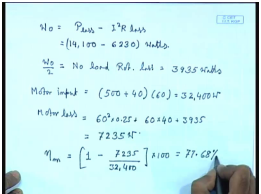So, the no load rotational loss of both the machine W 0 equal to p loss minus I square R loss. This comes to 14100 minus 6230 watts. So, W 0 by 2 which is no load rotational loss; this comes to 3935 watts. Motor power input equal to 500, the armature voltage plus 40 volt is the field voltage into 60. This comes to 32400 watts. Motor loss equal to armature circuit loss plus field circuit ohmic loss plus no load rotational losses; this comes to 7235.5 watts. So, motor efficiency nu m equal to 1 minus 7235 by 32400 into 100; this comes to 77.68 percent.

(Refer Slide Time: 53:21)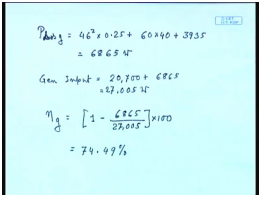So, total generator loss this is again 46 square into 0.25 plus 5. This comes to 6865 watts. So, generator input equal to generator output which was 20700 plus 6865 equal to 27005 watt. So, generator efficiency nu g equal to 1 minus 6865 divided by 27005 into 100. This comes to 74.49 percent.

Thank you.

Offer running on EduRev: Apply code STAYHOME200 to get INR 200 off on our premium plan EduRev Infinity!

61 docs|20 tests

,

,

,

,

,

,

,

,

,

,

,

,

,

,

,

,

,

,

,

,

,

;